### 15. THE LEAST-SQUARES METHOD

Until now we have been discussing the situation in which the experimental result is N events giving precise values x1, ... , xN where the xi may or may not, as the case may be, be all different.

From now on we shall confine our attention to the case of p measurements (not p events) at the points x1, ... , xp. The experimental results are (y1 ±1), ... ,(yp ±p). One such type of experiment is where each measurement consists of Ni events. Then yi = Ni and is Poisson-distributed withi = sqrt[Ni]. In this case the likelihood function is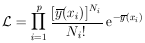and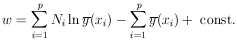We use the notation(i; x) for the curve that is to be fitted to the experimental points. The best-fit curve corresponds toi =i*. In this case of Poisson-distributed points, the solutions are obtained from the M simultaneous equations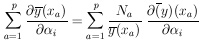If all the Ni >> 1, then it is a good approximation to assume each yi is Gaussian-distributed with standard deviationi. (It is better to use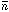i rather than Ni fori2 wherei can be obtained by integrating(x) over the ith interval.) Then one can use the famous least squares method.

The remainder of this section is devoted to the case in which yi are Gaussian-distributed with standard deviationsi. See Fig. 4. We shall now see that the least-squares method is mathematically equivalent to the maximum likelihood method. In this Gaussian case the likelihood function is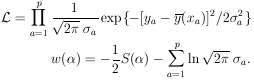(23)

where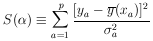(24)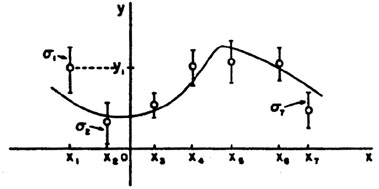Figure 4.(x) is a function of known shape to be fitted to the 7 experimental points.

The solutionsi =i* are given by minimizing S() (maximizing w):(25)

This minimum value of S is called S*, the least squares sum. The values ofi which minimize are called the least-squares solutions. Thus the maximum-likelihood and least-squares solutions are identical. According to Eq. (11), the least-squares errors are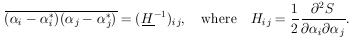Let us consider the special case in which(i; x) is linear in thei: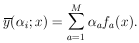(Do not confuse this f (x) with the f (x) on page 2.)

Then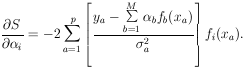(26)

Differentiating with respect toj gives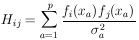(27)

Define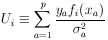(28)

Then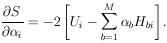In matrix notation the M simultaneous equations giving the least-squares solution are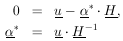(29)

is the solution for the*'s. The errors inare obtained using Eq. 11. To summarize: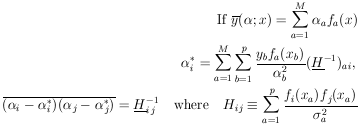(30)

Equation (30) is the complete procedure for calculating the least squares solutions and their errors. Note that even though this procedure is called curve-fitting it is never necessary to plot any curves. Quite often the complete experiment may be a combination of several experiments in which several different curves (all functions of thei) may be jointly fitted. Then the S-value is the sum over all the points on all the curves. Note that since w(*) decreases by ½ unit when one of thej has the value (i* ±j), the S-value must increase by one unit. That is,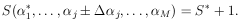Example 5 Linear regression with equal errors(x) is known to be of the form(x) =1 +2x. There are p experimental measurements (yj ±).Using Eq. (30) we have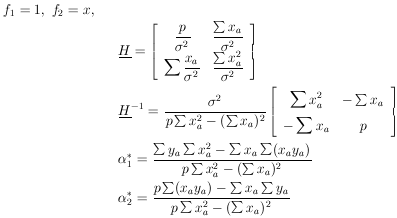These are the linear regression formulas which are programmed into many pocket calculators. They should not be used in those cases where thei are not all the same. If thei are all equal, the errors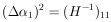or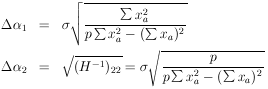Example 6 Quadratic regression with unequal errors

The curve to be fitted is known to be a parabola. There are four experimental points at x = - 0.6, - 0.2, 0.2, and 0.6. The experimental results are 5 ± 2, 3 ± 1, 5 ± 1, and 8 ± 2. Find the best-fit curve.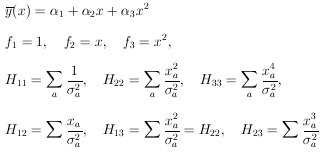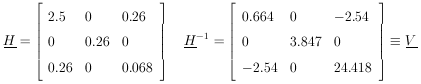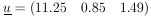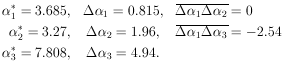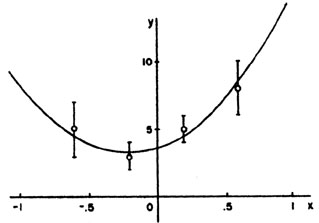Figure 5. This parabola is the least squares fit to the 4 experimental points in Example 6.

Example 7

In example 6 what is the best estimate of y at x = 1? What is the error of this estimate?

Solution: Putting x = 1 into the above equation gives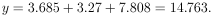y is obtained using Eq. 12.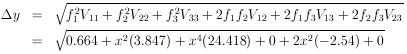Setting x = 1 gives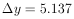So at x = 1, y = 14.763 ± 5.137.

Least Squares When the yi are Not Independent

Let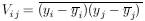be the error matrix-of the y measurements. Now we shall treat the more general case where the off diagonal elements need not be zero; i.e., the quantities yi are not independent. We see immediately from Eq. 11a that the log likelihood function is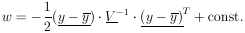The maximum likelihood solution is found by minimizing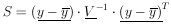whereGeneralized least squares sum